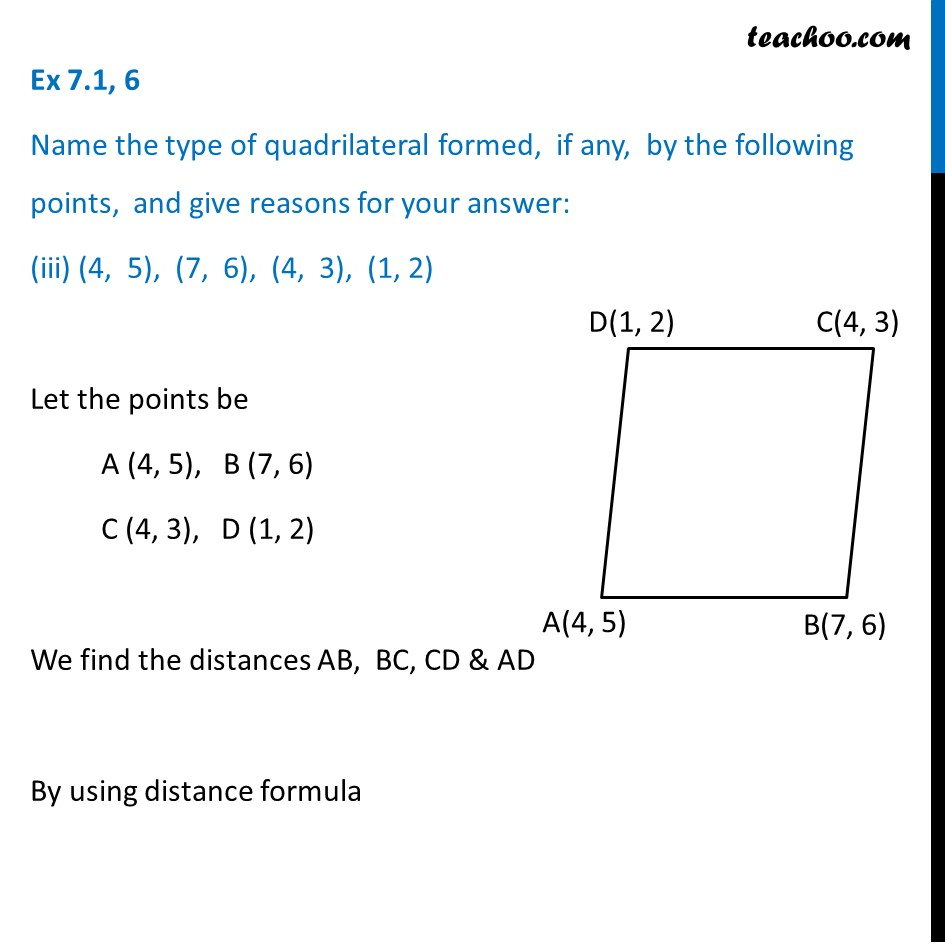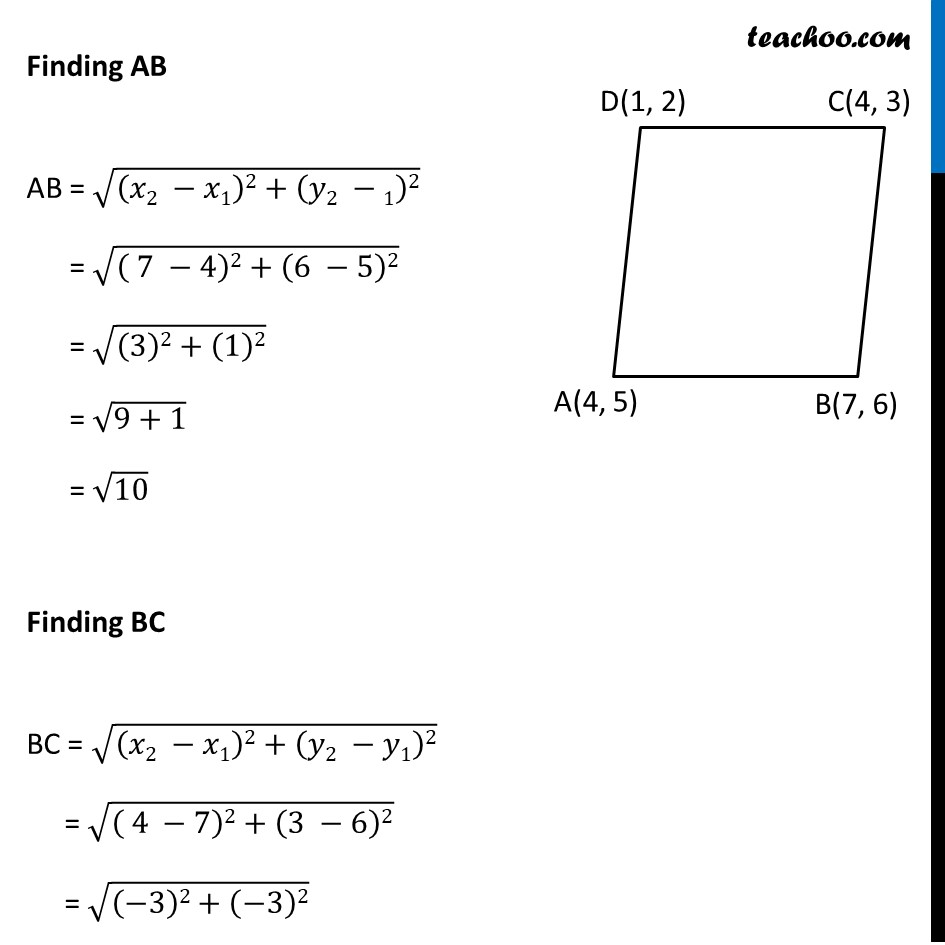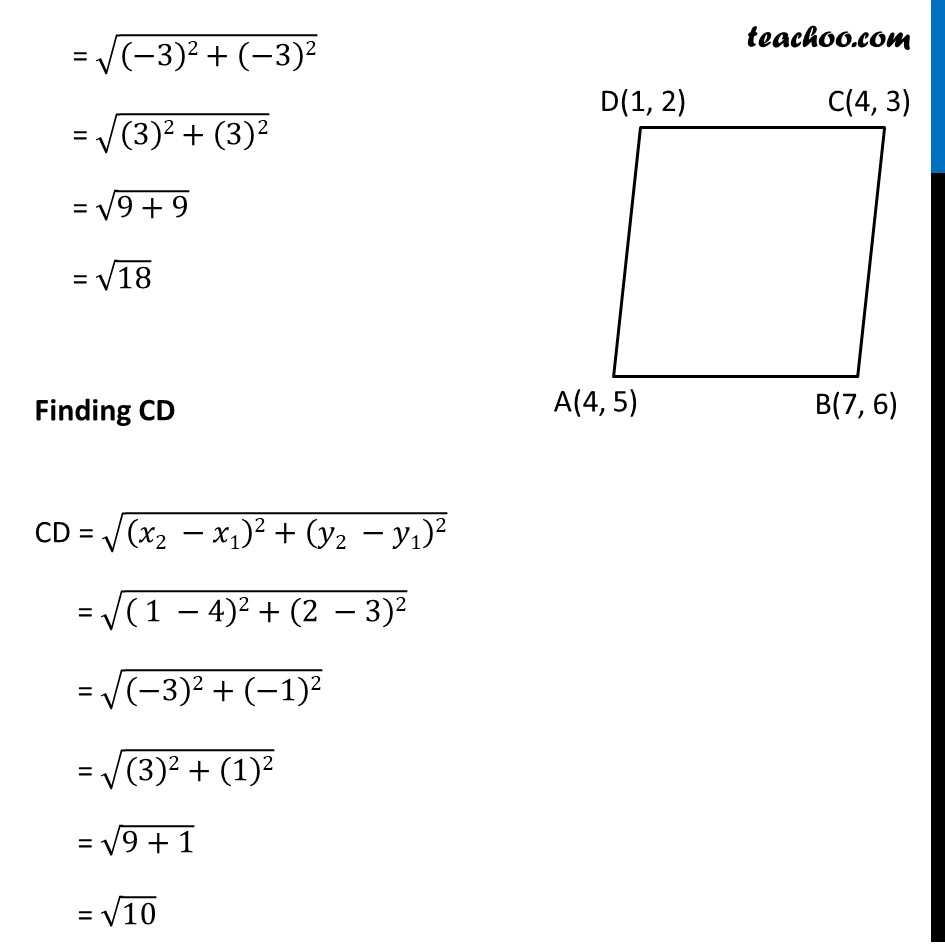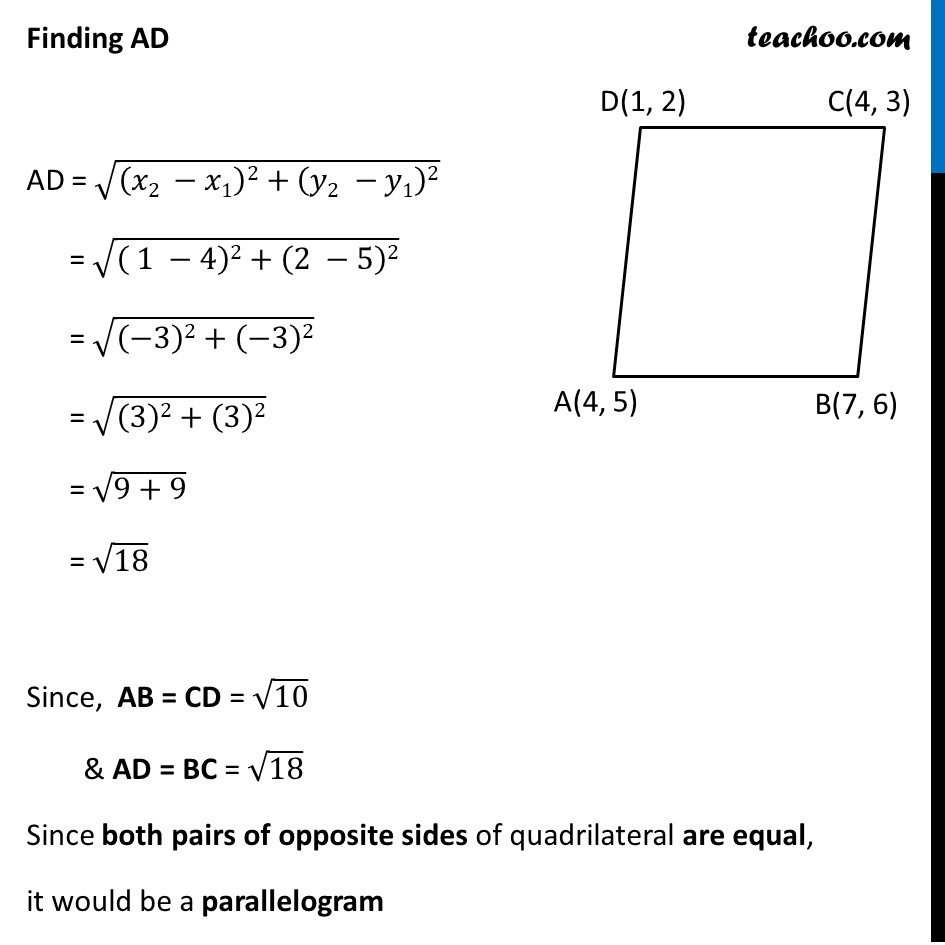Ex 7.1

Chapter 7 Class 10 Coordinate Geometry
Serial order wiseLearn in your speed, with individual attention - Teachoo Maths 1-on-1 Class

### Transcript

Ex 7.1, 6 Name the type of quadrilateral formed, if any, by the following points, and give reasons for your answer: (iii) (4, 5), (7, 6), (4, 3), (1, 2) Let the points be A (4, 5), B (7, 6) C (4, 3), D (1, 2) We find the distances AB, BC, CD & AD By using distance formula Finding AB AB = √((𝑥2 −𝑥1)2+(𝑦2 −1)2) = √(( 7 −4)2+(6 −5)2) = √((3)2+(1)2) = √(9+1) = √10 Finding BC BC = √((𝑥2 −𝑥1)2+(𝑦2 −𝑦1)2) = √(( 4 −7)2+(3 −6)2) = √((−3)2+(−3)2) = √((−3)2+(−3)2) = √((3)2+(3)2) = √(9+9) = √18 Finding CD CD = √((𝑥2 −𝑥1)2+(𝑦2 −𝑦1)2) = √(( 1 −4)2+(2 −3)2) = √((−3)2+(−1)2) = √((3)2+(1)2) = √(9+1) = √10 Finding AD AD = √((𝑥2 −𝑥1)2+(𝑦2 −𝑦1)2) = √(( 1 −4)2+(2 −5)2) = √((−3)2+(−3)2) = √((3)2+(3)2) = √(9+9) = √18 Since, AB = CD = √10 & AD = BC = √18 Since both pairs of opposite sides of quadrilateral are equal, it would be a parallelogram